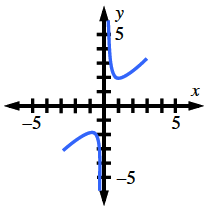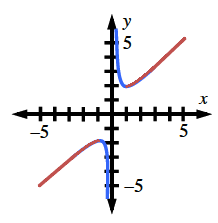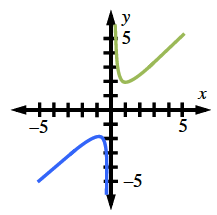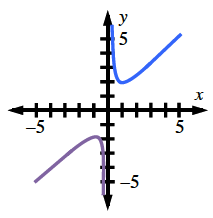### Home > PC > Chapter 7 > Lesson 7.3.1 > Problem7-92

7-92.

Graph the function $f(x) = \frac { x ^ { 2 } + 1 } { x }$ and answer the following questions.

Rational curve, with 2 sections, on either side of y-axis, left section increases from lower left to turning point (negative 1, comma negative 2), then decreases to negative infinity, right section decreases from infinity, to turning point (1, comma 2), increasing up & right.

1. On what intervals is the function increasing?

Function shaded on left section from lower left to turning point, & on right section from turning point to upper right.

2. Where is the function concave up?

Shading changed to section right of y-axis.

3. Where is the function concave down?

Shading change to section left of y-axis.

4. Is $f\left(x\right)$ even, odd, or neither? Justify your answer algebraically.

It is ODD. Show that: $−f\left(x\right) = f\left(−x\right)$